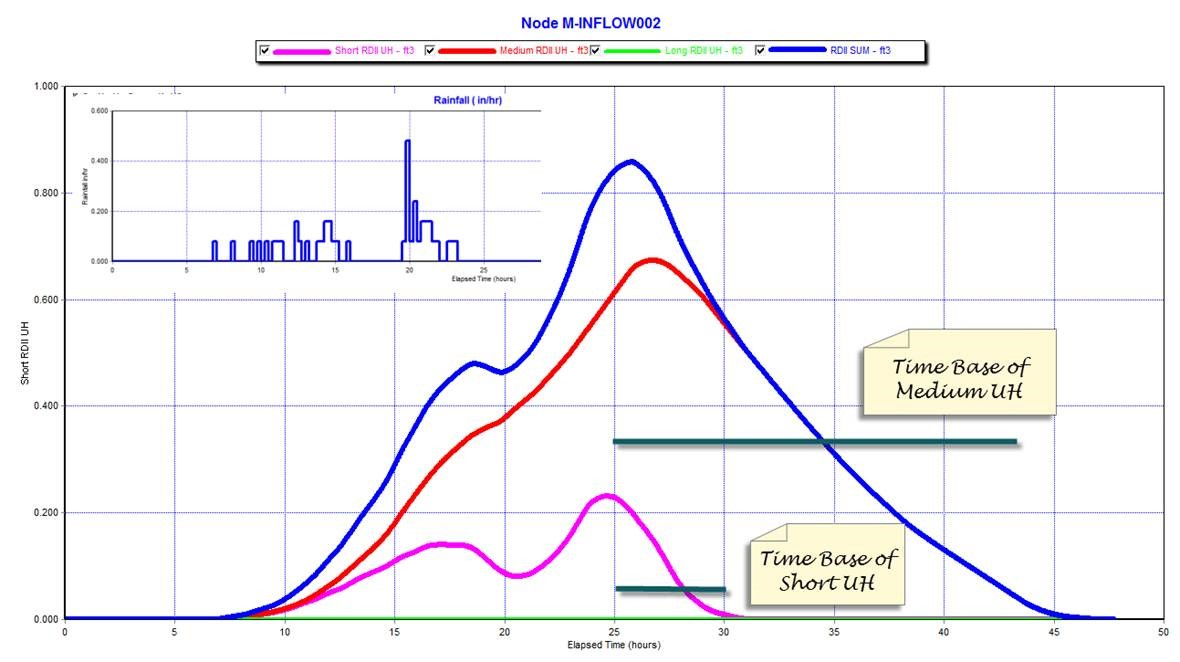# Convolution of the RDII UH from R, T and K in SWMM 5

Subject:  Convolution of the RDII UH from R, T and K in SWMM 5

The convolution uses the value of R and the Time Base to estimate the amount of Infiltration and Inflow in the Sewer Network.  The short, medium and long term UH‘s are estimated at each Wet Hydrology time step to make a smooth hydrograph out of the R, T and  K parameters of the Rainfall Dependent Infiltration and Infiltration Method (Figure 1).  The three UH‘s can be displaced as well if you use the RTK storage parameters (Figure 2)Figure 1.  The short, medium and long UH‘s are convoluted in SWMM 5 from the Rainfall Time Series.Figure 2.   The Initial Abstraction Depth can be used to shift the generated UH in time or reduce the peak flow and total volumes.

Translate »